• 转自：http://www.cnblogs.com/pegasus/archive/2011/05/20/2051780.html更复杂些的滤波算子一般是先利用高斯滤波来平滑，然后计算其1阶2阶微分。由于它们滤除高频低频，因此称为带通滤波器(band-pass filters)...
转自：http://www.cnblogs.com/pegasus/archive/2011/05/20/2051780.html更复杂些的滤波算子一般是先利用高斯滤波来平滑，然后计算其1阶和2阶微分。由于它们滤除高频和低频，因此称为带通滤波器(band-pass filters)。在介绍具体的带通滤波器前，先介绍必备的图像微分知识。1 一阶导数连续函数，其微分可表达为，或(1.1) 对于离散情况(图像)，其导数必须用差分方差来近似，有，前向差分 forward differencing                  (1.2)，中心差分 central differencing                     (1.3) 1)前向差分的Matlab实现2)中心差分的Matlab实现实例：技术图像x方向导数原图像                                                   x方向1阶导数2 图像梯度(Image Gradient)图像I的梯度定义为，其幅值为。出于计算性能考虑，幅值也可用来近似。 Matlab函数1)gradient：梯度计算2)quiver：以箭头形状绘制梯度。注意放大下面最右侧图可看到箭头，由于这里计算横竖两个方向的梯度，因此箭头方向都是水平或垂直的。实例：仍采用上面的原始图像梯度幅值                                   梯度幅值+梯度方向3 二阶导数对于一维函数，其二阶导数，即。它的差分函数为(3.1) 3.1 普拉斯算子(laplacian operator)3.1.2 概念拉普拉斯算子是n维欧式空间的一个二阶微分算子。它定义为两个梯度向量算子的内积(3.2) 其在二维空间上的公式为：(3.3) 对于1维离散情况，其二阶导数变为二阶差分1)首先，其一阶差分为2)因此，二阶差分为3)因此，1维拉普拉斯运算可以通过1维卷积核实现 对于2维离散情况(图像)，拉普拉斯算子是2个维上二阶差分的和(见式3.3)，其公式为：(3.4) 上式对应的卷积核为常用的拉普拉斯核有：3.1.2 应用拉普拉斯算子会突出像素值快速变化的区域，因此常用于边缘检测。Matlab里有两个函数1)del2计算公式：，2)fspecial：图像处理中一般利用Matlab函数fspecialh = fspecial('laplacian', alpha) returns a 3-by-3 filter approximating the shape of the two-dimensional Laplacian operator.The parameter alpha controls the shape of the Laplacian and must be in the range 0.0 to 1.0. The default value for alpha is 0.2.3.1.3 资源
展开全文• 更复杂些的滤波算子一般是先利用高斯滤波来平滑，...1 一阶导数连续函数，其微分可表达为 ，或(1.1)对于离散情况(图像)，其导数必须用差分方差来近似，有，前向差分 forwarddifferencing(1.2) ，中心差分 central...
更复杂些的滤波算子一般是先利用高斯滤波来平滑，然后计算其1阶和2阶微分。由于它们滤除高频和低频，因此称为带通滤波器(band-passfilters)。在介绍具体的带通滤波器前，先介绍必备的图像微分知识。1 一阶导数连续函数，其微分可表达为，或(1.1)对于离散情况(图像)，其导数必须用差分方差来近似，有，前向差分 forwarddifferencing (1.2)，中心差分 centraldifferencing (1.3)1)前向差分的Matlab实现function dimg = mipforwarddiff(img,direction)% MIPFORWARDDIFF     Finite difference calculations%%   DIMG = MIPFORWARDDIFF(IMG,DIRECTION)%%  Calculates the forward-difference for a given direction%  IMG       : input image%  DIRECTION : 'dx' or 'dy'%  DIMG      : resultant image%%   See also MIPCENTRALDIFF MIPBACKWARDDIFF MIPSECONDDERIV%   MIPSECONDPARTIALDERIV%   Omer Demirkaya, Musa Asyali, Prasana Shaoo, ... 9/1/06%   Medical Image Processing ToolboximgPad = padarray(img,[1 1],'symmetric','both');%将原图像的边界扩展[row,col] = size(imgPad);dimg = zeros(row,col);switch (direction)case 'dx',dimg(:,1:col-1) = imgPad(:,2:col)-imgPad(:,1:col-1);%x方向差分计算，case 'dy',dimg(1:row-1,:) = imgPad(2:row,:)-imgPad(1:row-1,:);otherwise, disp('Direction is unknown');end;dimg = dimg(2:end-1,2:end-1);01function dimg = mipforwarddiff(img,direction)02%MIPFORWARDDIFF Finite difference calculations03%04% DIMG =MIPFORWARDDIFF(IMG,DIRECTION)05%06% Calculates theforward-difference for a givendirection07% IMG : input image08% DIRECTION : 'dx' or 'dy'09% DIMG : resultant image10%11% See alsoMIPCENTRALDIFF MIPBACKWARDDIFF MIPSECONDDERIV12% MIPSECONDPARTIALDERIV1314% Omer Demirkaya,Musa Asyali, Prasana Shaoo, ... 9/1/0615% Medical ImageProcessing Toolbox1617imgPad = padarray(img,[1 1],'symmetric','both');%将原图像的边界扩展18[row,col] = size(imgPad);19dimg = zeros(row,col);20switch(direction)21case'dx',22dimg(:,1:col-1)= imgPad(:,2:col)-imgPad(:,1:col-1);%x方向差分计算，23case'dy',24dimg(1:row-1,:)= imgPad(2:row,:)-imgPad(1:row-1,:);25otherwise, disp('Direction is unknown');26end;27dimg = dimg(2:end-1,2:end-1);2)中心差分的Matlab实现function dimg = mipcentraldiff(img,direction)% MIPCENTRALDIFF     Finite difference calculations%%   DIMG = MIPCENTRALDIFF(IMG,DIRECTION)%%  Calculates the central-difference for a given direction%  IMG       : input image%  DIRECTION : 'dx' or 'dy'%  DIMG      : resultant image%%   See also MIPFORWARDDIFF MIPBACKWARDDIFF MIPSECONDDERIV%   MIPSECONDPARTIALDERIV%   Omer Demirkaya, Musa Asyali, Prasana Shaoo, ... 9/1/06%   Medical Image Processing Toolboximg = padarray(img,[1 1],'symmetric','both');[row,col] = size(img);dimg = zeros(row,col);switch (direction)case 'dx',dimg(:,2:col-1) = (img(:,3:col)-img(:,1:col-2))/2;case 'dy',dimg(2:row-1,:) = (img(3:row,:)-img(1:row-2,:))/2;otherwise,disp('Direction is unknown');enddimg = dimg(2:end-1,2:end-1);1实例：技术图像x方向导数I = imread('coins.png'); figure; imshow(I);Id = mipforwarddiff(I,'dx'); figure, imshow(Id);1I = imread('coins.png'); figure;imshow(I);2Id = mipforwarddiff(I,'dx'); figure,imshow(Id);原图像 x方向1阶导数2 图像梯度(Image Gradient)图像I的梯度定义为，其幅值为。出于计算性能考虑，幅值也可用来近似。Matlab函数1)gradient：梯度计算2)quiver：以箭头形状绘制梯度。注意放大下面最右侧图可看到箭头，由于这里计算横竖两个方向的梯度，因此箭头方向都是水平或垂直的。实例：仍采用上面的原始图像I = double(imread('coins.png'));[dx,dy]=gradient(I);magnitudeI=sqrt(dx.^2+dy.^2);figure;imagesc(magnitudeI);colormap(gray);%梯度幅值hold on;quiver(dx,dy);%叠加梯度方向1I = double(imread('coins.png'));2[dx,dy]=gradient(I);3magnitudeI=sqrt(dx.^2+dy.^2);4figure;imagesc(magnitudeI);colormap(gray);%梯度幅值5hold on;quiver(dx,dy);%叠加梯度方向梯度幅值 梯度幅值+梯度方向3 二阶导数对于一维函数，其二阶导数，即。它的差分函数为(3.1)3.1 普拉斯算子(laplacian operator)3.1.2 概念拉普拉斯算子是n维欧式空间的一个二阶微分算子。它定义为两个梯度向量算子的内积(3.2)其在二维空间上的公式为：(3.3)对于1维离散情况，其二阶导数变为二阶差分1)首先，其一阶差分为2)因此，二阶差分为3)因此，1维拉普拉斯运算可以通过1维卷积核实现对于2维离散情况(图像)，拉普拉斯算子是2个维上二阶差分的和(见式3.3)，其公式为：(3.4)上式对应的卷积核为常用的拉普拉斯核有：3.1.2 应用拉普拉斯算子会突出像素值快速变化的区域，因此常用于边缘检测。Matlab里有两个函数1)del2计算公式：，2)fspecial：图像处理中一般利用Matlab函数fspecialh = fspecial('laplacian', alpha) returns a 3-by-3 filterapproximating the shape of the two-dimensional Laplacianoperator.The parameter alpha controls the shape of the Laplacian and must bein the range 0.0 to 1.0. The default value for alpha is 0.2.
展开全文• 如果图像灰度变化剧烈，进行一阶微分则会形成一个局部的极值，由数学上的知识，对图像进行二阶微分则会形成一个过零点，并且在零点两边产生一个波峰波谷，我们要设定一个阈值，检测到这个过零点，如下图所示： ...
目录

一、概述

二、拉普拉斯算子(Laplace)

三、高斯——拉普拉斯算子(LOG)

四、Canny

1. 写在前面

2. Canny边缘检测算法的发展历史

3. Canny边缘检测算法的处理流程

3.1 高斯平滑滤波

3.2 计算梯度强度和方向

3.3 非极大值抑制

3.4 双阈值检测

3.5 抑制孤立低阈值点

一、概述

如果图像灰度变化剧烈，进行一阶微分则会形成一个局部的极值，由数学上的知识，对图像进行二阶微分则会形成一个过零点，并且在零点两边产生一个波峰和波谷，我们要设定一个阈值，检测到这个过零点，如下图所示：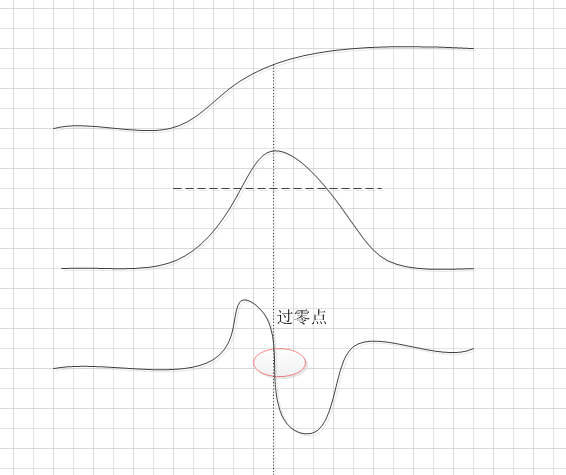带来了两个好处：

1. 二阶微分关心的是图像灰度的突变而不强调灰度缓慢变化的区域，对边缘的定位能力更强。

2. Laplace算子是各项同性的，即具有旋转不变性（后面会证明），在一阶微分里，我们是用|dx|+|dy|来近似一个点的梯度    的，当图像旋转一个角度时，这个值就变化了，但对于Laplace算子来说不管图像怎么旋转，得到的响应是一样的。

一个注意点：

我们检测的必须是“过零点“，而不单单是零点，也就是要保证这个被选中的点一定要是局部极值点。比如下面这个例子，上面的曲线是图像空间，虚线处的点并不是图像的局部极值点，但求二阶导的时候确实是零点。再比如图像灰度的平坦区域，不管是一阶导还是二阶导都是0，它们显然不是我们要的点：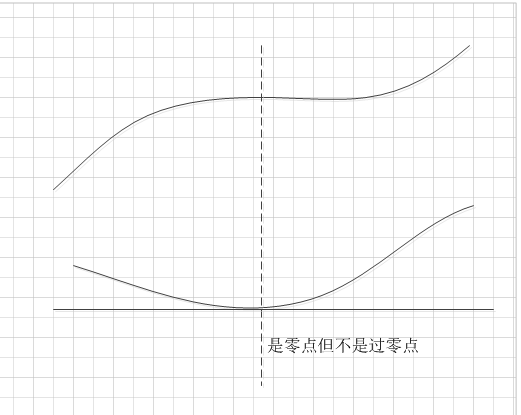过零点的确定：

以p为中心的一个3*3领域，p点为过零点意味着至少有两个相对的领域像素的符号不同。有四种要检测的情况：左/右、上/下，和两个对角。如果g(x,y)的值与一个阈值比较（一种通用的方法），那么不仅要求相对领域的符号不同，数值差的绝对值要超过这个阈值，这时p称为一个过零点像素。

二、拉普拉斯算子(Laplace)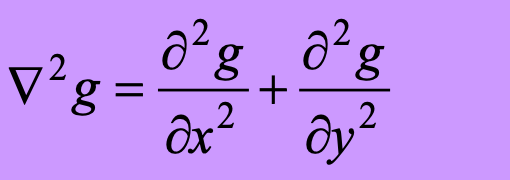用差分近似微分：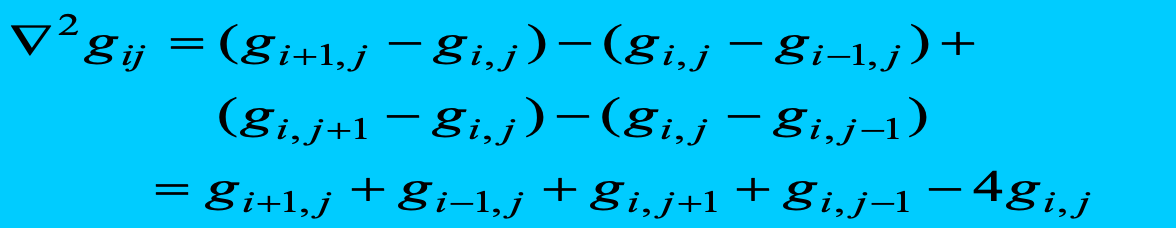模版为：用卷积核进行掩膜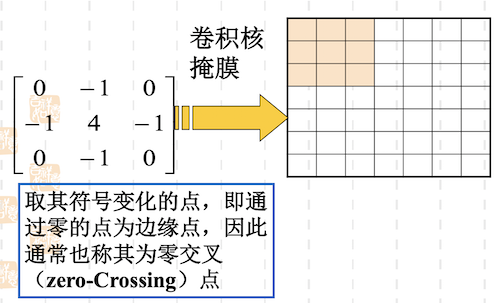掩膜后的结果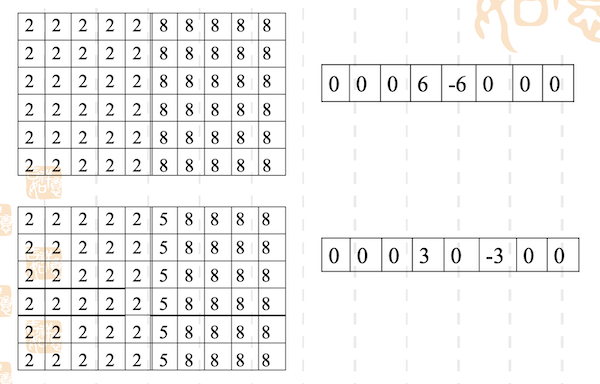三、高斯——拉普拉斯算子(LOG)

首先用高斯函数先进行低通滤波，然后利用拉普拉斯算子进行高通滤波并提取零交叉点：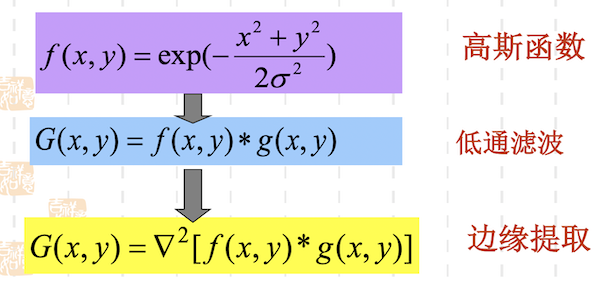微分算子与卷积算子的次序可以交换。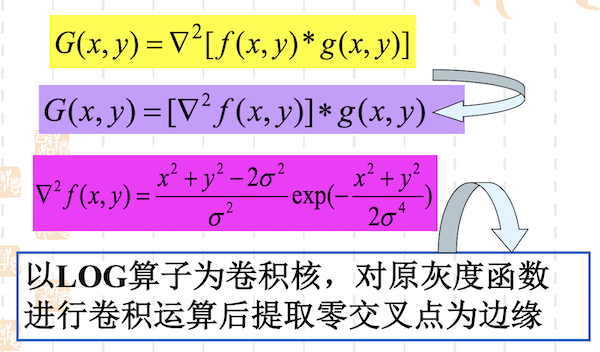基本特征：

•平滑滤波器是高斯滤波器．

•采用拉普拉斯算子计算二阶导数．

•边缘检测判据是二阶导数零交叉点并对应一阶导数的较大峰值．

•使用线性内插方法在子像素分辨率水平上估计边缘的位置．

下面是一个5x5的高斯拉普拉斯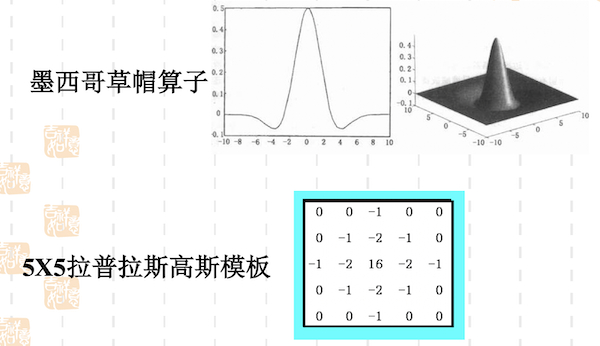四、Canny

1. 写在前面

最近在做边缘检测方面的一些工作，在网络上也找了很多有用的资料，感谢那些积极分享知识的先辈们，自己在理解Canny边缘检测算法的过程中也走了一些弯路，在编程实现的过程中，也遇到了一个让我怀疑人生的BUG（日了狗狗）。就此写下此文，作为后记，也希望此篇文章可以帮助那些在理解Canny算法的道路上暂入迷途的童鞋。废话少说，上干货。

2. Canny边缘检测算法的发展历史

Canny边缘检测于1986年由JOHN CANNY首次在论文《A Computational Approach to Edge Detection》中提出，就此拉开了Canny边缘检测算法的序幕。

Canny边缘检测是从不同视觉对象中提取有用的结构信息并大大减少要处理的数据量的一种技术，目前已广泛应用于各种计算机视觉系统。Canny发现，在不同视觉系统上对边缘检测的要求较为类似，因此，可以实现一种具有广泛应用意义的边缘检测技术。边缘检测的一般标准包括：

1)        以低的错误率检测边缘，也即意味着需要尽可能准确的捕获图像中尽可能多的边缘。

2)        检测到的边缘应精确定位在真实边缘的中心。

3)        图像中给定的边缘应只被标记一次，并且在可能的情况下，图像的噪声不应产生假的边缘。

为了满足这些要求，Canny使用了变分法。Canny检测器中的最优函数使用四个指数项的和来描述，它可以由高斯函数的一阶导数来近似。

在目前常用的边缘检测方法中，Canny边缘检测算法是具有严格定义的，可以提供良好可靠检测的方法之一。由于它具有满足边缘检测的三个标准和实现过程简单的优势，成为边缘检测最流行的算法之一。

3. Canny边缘检测算法的处理流程

Canny边缘检测算法可以分为以下5个步骤：

1)        使用高斯滤波器，以平滑图像，滤除噪声。

2)        计算图像中每个像素点的梯度强度和方向。

3)        应用非极大值（Non-Maximum Suppression）抑制，以消除边缘检测带来的杂散响应。

4)        应用双阈值（Double-Threshold）检测来确定真实的和潜在的边缘。

5)        通过抑制孤立的弱边缘最终完成边缘检测。

下面详细介绍每一步的实现思路。

3.1 高斯平滑滤波

为了尽可能减少噪声对边缘检测结果的影响，所以必须滤除噪声以防止由噪声引起的错误检测。为了平滑图像，使用高斯滤波器与图像进行卷积，该步骤将平滑图像，以减少边缘检测器上明显的噪声影响。大小为(2k+1)x(2k+1)的高斯滤波器核的生成方程式由下式给出：下面是一个sigma = 1.4，尺寸为3x3的高斯卷积核的例子（需要注意归一化）：若图像中一个3x3的窗口为A，要滤波的像素点为e，则经过高斯滤波之后，像素点e的亮度值为：其中*为卷积符号，sum表示矩阵中所有元素相加求和。

3.2 计算梯度强度和方向

图像中的边缘可以指向各个方向，因此Canny算法使用四个算子来检测图像中的水平、垂直和对角边缘。边缘检测的算子（如Roberts，Prewitt，Sobel等）返回水平Gx和垂直Gy方向的一阶导数值，由此便可以确定像素点的梯度G和方向theta 。其中G为梯度强度， theta表示梯度方向，arctan为反正切函数。下面以Sobel算子为例讲述如何计算梯度强度和方向。

x和y方向的Sobel算子分别为：其中Sx表示x方向的Sobel算子，用于检测y方向的边缘； Sy表示y方向的Sobel算子，用于检测x方向的边缘（边缘方向和梯度方向垂直）。在直角坐标系中，Sobel算子的方向如下图所示。图3-1 Sobel算子的方向

若图像中一个3x3的窗口为A，要计算梯度的像素点为e，则和Sobel算子进行卷积之后，像素点e在x和y方向的梯度值分别为：其中*为卷积符号，sum表示矩阵中所有元素相加求和。根据公式（3-2）便可以计算出像素点e的梯度和方向。

3.3 非极大值抑制

非极大值抑制是一种边缘稀疏技术，非极大值抑制的作用在于“瘦”边。对图像进行梯度计算后，仅仅基于梯度值提取的边缘仍然很模糊。对于标准3，对边缘有且应当只有一个准确的响应。而非极大值抑制则可以帮助将局部最大值之外的所有梯度值抑制为0，对梯度图像中每个像素进行非极大值抑制的算法是：

1)        将当前像素的梯度强度与沿正负梯度方向上的两个像素进行比较。

2)        如果当前像素的梯度强度与另外两个像素相比最大，则该像素点保留为边缘点，否则该像素点将被抑制。

通常为了更加精确的计算，在跨越梯度方向的两个相邻像素之间使用线性插值来得到要比较的像素梯度，现举例如下：图3-2 梯度方向分割

如图3-2所示，将梯度分为8个方向，分别为E、NE、N、NW、W、SW、S、SE，其中0代表00~45o,1代表450~90o，2代表-900~-45o，3代表-450~0o。像素点P的梯度方向为theta，则像素点P1和P2的梯度线性插值为：因此非极大值抑制的伪代码描写如下：需要注意的是，如何标志方向并不重要，重要的是梯度方向的计算要和梯度算子的选取保持一致。

3.4 双阈值检测

在施加非极大值抑制之后，剩余的像素可以更准确地表示图像中的实际边缘。然而，仍然存在由于噪声和颜色变化引起的一些边缘像素。为了解决这些杂散响应，必须用弱梯度值过滤边缘像素，并保留具有高梯度值的边缘像素，可以通过选择高低阈值来实现。如果边缘像素的梯度值高于高阈值，则将其标记为强边缘像素；如果边缘像素的梯度值小于高阈值并且大于低阈值，则将其标记为弱边缘像素；如果边缘像素的梯度值小于低阈值，则会被抑制。阈值的选择取决于给定输入图像的内容。

双阈值检测的伪代码描写如下：3.5 抑制孤立低阈值点

到目前为止，被划分为强边缘的像素点已经被确定为边缘，因为它们是从图像中的真实边缘中提取出来的。然而，对于弱边缘像素，将会有一些争论，因为这些像素可以从真实边缘提取也可以是因噪声或颜色变化引起的。为了获得准确的结果，应该抑制由后者引起的弱边缘。通常，由真实边缘引起的弱边缘像素将连接到强边缘像素，而噪声响应未连接。为了跟踪边缘连接，通过查看弱边缘像素及其8个邻域像素，只要其中一个为强边缘像素，则该弱边缘点就可以保留为真实的边缘。

抑制孤立边缘点的伪代码描述如下：4 总结

通过以上5个步骤即可完成基于Canny算法的边缘提取，图5-1是该算法的检测效果图，希望对大家有所帮助。图5-1 Canny边缘检测效果


展开全文• 在本文中，我们考虑了拟线性双曲型偏微分方程初值问题（IVP）数值解的特征数值方法，以及差分方案中央时间中心空间（CTCS），Crank-Nicolson方案，ω方案一维齐次波动方程初值和边值问题数值解的特征方法。...
• 常常采用基于一阶或二阶微分的锐化滤波器实现图像的锐化处理。 一阶微分 一阶微分是通过梯度法来实现的。对于图像f(i,j)，它在点(i,j)处的梯度是一个矢量，定义为： 利用差分法近似上述公式，得到： 为了便于编程...
图象锐化
建议先查看图像平滑

锐化处理的主要目的是突出图像中的细节或者增强被模糊了的细节，这种模糊不是由于错误操作，就是特殊图像获取方法的固有影响。图像均值滤波器可以使图像变模糊，是因为均值处理与积分相类似，因此可以对其进行逆运算（如微分运算）就可以使图像变得清晰。
常常采用基于一阶或二阶微分的锐化滤波器实现图像的锐化处理。
一阶微分
一阶微分是通过梯度法来实现的。对于图像f(i,j)，它在点(i,j)处的梯度是一个矢量，定义为：利用差分法近似上述公式，得到：为了便于编程和提高运算，可进一步简化为：利用差分运算时，图像的第一行和第一列的像素的梯度无法求得，一般用后一行或后一列的梯度值近似代替。微分运算可以增强图像高频分量（边缘等细节），但是仅仅微分处理后的图像非常暗，因此既要增强图像边缘，又要保持目标物体的内部灰度不变，常采用给边缘规定一个特定的灰度级的方法来完成梯度锐化处理。公式为：La为一指定的灰度值，它将边界的灰度值统一化，这样可以使其边界更加清晰明显。该方法基本上不破坏图像的背景，又可找到边缘，并根据需要增强边缘。
基于二阶微分的锐化滤波器
基于二阶微分的锐化滤波器，即拉普拉斯增强算子。一个二元图像函数f(x, y)的拉普拉斯变换定义为：由于拉普拉斯是一种微分算子，它的应用强调图像中灰度的突变及降低灰度慢变化的区域，这将产生一幅把图像中的浅灰色边线和突变点叠加到暗背景中的图像。将原始图像和拉普拉斯图像叠加在一起的简单方法可以保护拉普拉斯锐化处理的效果，同时又能复原背景信息。这种方法可表示为：OpenCV实现
对去噪后的图像进行锐化，分别采用一阶微分和二阶微分，比较锐化效果。一阶微分
罗伯特交叉算子
// 梯度锐化函数
//         -1   1
//  -1
//   1
Mat GradientSharpening(const Mat& src, Mat& dst) {
if (!src.data) return src;
for (int i = 0; i < src.rows; ++i)
for (int j = 0; j < src.cols; ++j) {
int a, b, c;
if (i < src.rows - 1 && j < src.cols - 1) {

//罗伯特交叉算子
a = abs(src.at<Vec3b>(i + 1, j ) - src.at<Vec3b>(i, j)) + abs(src.at<Vec3b>(i , j + 1) - src.at<Vec3b>(i, j));
b = abs(src.at<Vec3b>(i + 1, j ) - src.at<Vec3b>(i, j)) + abs(src.at<Vec3b>(i , j + 1) - src.at<Vec3b>(i, j));
c = abs(src.at<Vec3b>(i + 1, j ) - src.at<Vec3b>(i, j)) + abs(src.at<Vec3b>(i , j + 1) - src.at<Vec3b>(i, j));

//加上原来的颜色值，实现锐化增强
a = a + src.at<Vec3b>(i, j);
b = b + src.at<Vec3b>(i, j);
c = c + src.at<Vec3b>(i, j);
}
else {
//无法处理的直接赋值原来的颜色值
a = src.at<Vec3b>(i, j);
b = src.at<Vec3b>(i, j);
c = src.at<Vec3b>(i, j);
}
//颜色值 超过255，为255
if (a > 255 || b > 255 || b > 255) {

dst.at<Vec3b>(i, j) = src.at<Vec3b>(i, j);
dst.at<Vec3b>(i, j) = src.at<Vec3b>(i, j);
dst.at<Vec3b>(i, j) = src.at<Vec3b>(i, j);
}
else {
dst.at<Vec3b>(i, j) = a;
dst.at<Vec3b>(i, j) = b;
dst.at<Vec3b>(i, j) = c;
}
}
return dst;
}


二阶微分
// 拉普拉斯锐化函数
// 0  -1  0
// -1  4  -1
// 0  -1  0
Mat LaplacianSharpening(const Mat& src, Mat& dst) {
if (!src.data) return src;
Mat img(src.size(), src.type());
for (int i = 0; i < src.rows; ++i)
for (int j = 0; j < src.cols; ++j) {
int a, b, c;
if (i > 1 && j > 1 && i < src.rows - 1  && j < src.cols - 1) {
//5 是因为直接加上了原来的颜色值
a = abs(4 * src.at<Vec3b>(i, j) - src.at<Vec3b>(i - 1, j) - src.at<Vec3b>(i + 1, j) - src.at<Vec3b>(i, j - 1) - src.at<Vec3b>(i, j + 1) );
b = abs(4 * src.at<Vec3b>(i, j) - src.at<Vec3b>(i - 1, j) - src.at<Vec3b>(i + 1, j) - src.at<Vec3b>(i, j - 1) - src.at<Vec3b>(i, j + 1) );
c = abs(4 * src.at<Vec3b>(i, j) - src.at<Vec3b>(i - 1, j) - src.at<Vec3b>(i + 1, j) - src.at<Vec3b>(i, j - 1) - src.at<Vec3b>(i, j + 1) );

a += src.at<Vec3b>(i, j);
b += src.at<Vec3b>(i, j);
c += src.at<Vec3b>(i, j);
}
else {
a = src.at<Vec3b>(i, j);
b = src.at<Vec3b>(i, j);
c = src.at<Vec3b>(i, j);
}
if (a > 255 || b > 255 || b > 255 ) {
dst.at<Vec3b>(i, j) = src.at<Vec3b>(i, j);
dst.at<Vec3b>(i, j) = src.at<Vec3b>(i, j);
dst.at<Vec3b>(i, j) = src.at<Vec3b>(i, j);
}
else {
dst.at<Vec3b>(i, j) = a;
dst.at<Vec3b>(i, j) = b;
dst.at<Vec3b>(i, j) = c;
}
}
return dst;
}

所有代码
包含了前一篇的代码
#include<opencv2\opencv.hpp>
#include<iostream>

using namespace cv;
using namespace std;

//2021.4.25
//Solye

//求均值
uchar getAverage(Mat src,int i, int j,int way) {
uchar newData;
newData = (
src.at<Vec3b>(i-1, j)[way] + src.at<Vec3b>(i - 1, j)[way] + src.at<Vec3b>(i - 1, j+1)[way] +
src.at<Vec3b>(i, j - 1)[way] +src.at<Vec3b>(i , j)[way] + src.at<Vec3b>(i, j + 1)[way] +
src.at<Vec3b>(i + 1, j - 1)[way] + src.at<Vec3b>(i+1, j )[way] + src.at<Vec3b>(i + 1, j+1)[way]
) / 9;

return newData;
}

//均值滤波
Mat AverageFilter(const Mat& src, Mat& dst) {
if (!src.data) return src;

for (int i = 0; i < src.rows; ++i)
for (int j = 0; j < src.cols; ++j) {

if (i >= 1 && j >= 1 && i < src.rows - 1 && j < src.cols - 1) {
dst.at<Vec3b>(i, j) = getAverage(src, i, j, 0);
dst.at<Vec3b>(i, j) = getAverage(src, i, j, 1);
dst.at<Vec3b>(i, j) = getAverage(src, i, j, 2);
}
else {

dst.at<Vec3b>(i, j) = src.at<Vec3b>(i, j);
dst.at<Vec3b>(i, j) = src.at<Vec3b>(i, j);
dst.at<Vec3b>(i, j) = src.at<Vec3b>(i, j);
}
}
return dst;
}
//求中值
uchar getMedian(Mat src, int a, int b, int way) {
uchar newArray;
newArray = src.at<Vec3b>(a - 1, b - 1)[way];
newArray = src.at<Vec3b>(a - 1, b )[way];
newArray = src.at<Vec3b>(a - 1, b + 1)[way];
newArray = src.at<Vec3b>(a , b -1)[way];
newArray = src.at<Vec3b>(a , b )[way];
newArray = src.at<Vec3b>(a , b + 1)[way];
newArray = src.at<Vec3b>(a + 1, b -1)[way];
newArray = src.at<Vec3b>(a + 1, b)[way];
newArray = src.at<Vec3b>(a + 1, b+1)[way];
int len = (int)sizeof(newArray) / sizeof(uchar);
uchar temp;
for (int i = 0; i < len - 1; i++)
for (int j = 0; j < len - 1 - i; j++)
if (newArray[j] > newArray[j + 1])
{
temp = newArray[j];
newArray[j] = newArray[j + 1];
newArray[j + 1] = temp;
}

return  newArray;
}

//中值滤波
Mat MedianFilter(const Mat& src, Mat& dst) {
if (!src.data) return src;
for (int i = 0; i < src.rows; ++i) {
for (int j = 0; j < src.cols; ++j) {
if (i >= 1 && j >= 1 && i < src.rows - 1 && j < src.cols - 1) {
dst.at<Vec3b>(i, j) = getMedian(src, i, j, 0);
dst.at<Vec3b>(i, j) = getMedian(src, i, j, 1);
dst.at<Vec3b>(i, j) = getMedian(src, i, j, 2);
}
else {
dst.at<Vec3b>(i, j) = src.at<Vec3b>(i, j);
dst.at<Vec3b>(i, j) = src.at<Vec3b>(i, j);
dst.at<Vec3b>(i, j) = src.at<Vec3b>(i, j);
}
}
}
return dst;
}

// 梯度锐化函数
Mat GradientSharpening(const Mat& src, Mat& dst) {
if (!src.data) return src;
for (int i = 0; i < src.rows; ++i)
for (int j = 0; j < src.cols; ++j) {
int a, b, c;
if (i < src.rows - 1 && j < src.cols - 1) {

//罗伯特交叉算子
a = abs(src.at<Vec3b>(i + 1, j ) - src.at<Vec3b>(i, j)) + abs(src.at<Vec3b>(i , j + 1) - src.at<Vec3b>(i, j));
b = abs(src.at<Vec3b>(i + 1, j ) - src.at<Vec3b>(i, j)) + abs(src.at<Vec3b>(i , j + 1) - src.at<Vec3b>(i, j));
c = abs(src.at<Vec3b>(i + 1, j ) - src.at<Vec3b>(i, j)) + abs(src.at<Vec3b>(i , j + 1) - src.at<Vec3b>(i, j));

//加上原来的颜色值，实现锐化增强
a = a + src.at<Vec3b>(i, j);
b = b + src.at<Vec3b>(i, j);
c = c + src.at<Vec3b>(i, j);
}
else {
//无法处理的直接赋值原来的颜色值
a = src.at<Vec3b>(i, j);
b = src.at<Vec3b>(i, j);
c = src.at<Vec3b>(i, j);
}
//颜色值 超过255，为255
if (a > 255 || b > 255 || b > 255) {

dst.at<Vec3b>(i, j) = src.at<Vec3b>(i, j);
dst.at<Vec3b>(i, j) = src.at<Vec3b>(i, j);
dst.at<Vec3b>(i, j) = src.at<Vec3b>(i, j);
}
else {
dst.at<Vec3b>(i, j) = a;
dst.at<Vec3b>(i, j) = b;
dst.at<Vec3b>(i, j) = c;
}
}
return dst;
}

// 拉普拉斯锐化函数
// 0  -1  0
// -1  4  -1
// 0  -1  0
Mat LaplacianSharpening(const Mat& src, Mat& dst) {
if (!src.data) return src;
Mat img(src.size(), src.type());
for (int i = 0; i < src.rows; ++i)
for (int j = 0; j < src.cols; ++j) {
int a, b, c;
if (i > 1 && j > 1 && i < src.rows - 1  && j < src.cols - 1) {
//5 是因为直接加上了原来的颜色值
a = abs(4 * src.at<Vec3b>(i, j) - src.at<Vec3b>(i - 1, j) - src.at<Vec3b>(i + 1, j) - src.at<Vec3b>(i, j - 1) - src.at<Vec3b>(i, j + 1) );
b = abs(4 * src.at<Vec3b>(i, j) - src.at<Vec3b>(i - 1, j) - src.at<Vec3b>(i + 1, j) - src.at<Vec3b>(i, j - 1) - src.at<Vec3b>(i, j + 1) );
c = abs(4 * src.at<Vec3b>(i, j) - src.at<Vec3b>(i - 1, j) - src.at<Vec3b>(i + 1, j) - src.at<Vec3b>(i, j - 1) - src.at<Vec3b>(i, j + 1) );

a += src.at<Vec3b>(i, j);
b += src.at<Vec3b>(i, j);
c += src.at<Vec3b>(i, j);
}
else {
a = src.at<Vec3b>(i, j);
b = src.at<Vec3b>(i, j);
c = src.at<Vec3b>(i, j);
}
if (a > 255 || b > 255 || b > 255 ) {
dst.at<Vec3b>(i, j) = src.at<Vec3b>(i, j);
dst.at<Vec3b>(i, j) = src.at<Vec3b>(i, j);
dst.at<Vec3b>(i, j) = src.at<Vec3b>(i, j);
}
else {
dst.at<Vec3b>(i, j) = a;
dst.at<Vec3b>(i, j) = b;
dst.at<Vec3b>(i, j) = c;
}
}
return dst;
}

int main()
{
Mat averageFilter;
Mat medianFilter;
Mat laplacianSharpening;

averageFilter.create(src.rows, src.cols, src.type());
medianFilter.create(src.rows, src.cols, src.type());
laplacianSharpening.create(src.rows, src.cols, src.type());

imshow("原图", src);
imshow("均值滤波", AverageFilter(src, averageFilter));
imshow("中值滤波", MedianFilter(src,medianFilter));
imshow("拉普拉斯增强算子锐化", LaplacianSharpening(medianFilter, laplacianSharpening));

return waitKey(0);

}



展开全文opencv 计算机视觉 边缘检测
• 微分方程的数值解法主要包括两大类：有限差分有限单元法。这里主要介绍有限单元法。 However，对于一个只学过微积分矩阵论的工科生来说，要了解有限元法的数学原理还是有些困难，所以这里重点是介绍有限元法...计算数学
• 电子散斑干涉技术(ESPI)中，基于偏微分方程(PDE)的滤波模型是一种重要的滤波方法。...九点差分和中心差分格式需要使用均值滤波做进一步的处理，中心差分格式处理速度最快，高阶差分格式次之，九点差分格式则最慢。
• 差异完成 通过有限差分法在一维二维中使用有限差分法求解二阶微分方程 我们使用库来具有操作矩阵的基本功能，两个矩阵的乘积，逆数... 实施一组测试以验证算法Java
• 在所有有限差分表达式中，系数之为...为了解决这个矛盾，我们可以采取以下措施：1 使用双精度浮点数运算2 采用精确度至少为的有限差分公式例如，用中心差分法计算 在 处的二阶导数。取不同的 值以及精度为 ，手...
• 交错网格微积分 StaggeredGridCalculus是一个Julia包，为矢量场... 向量场在交错的有限差分网格（也称为Yee网格）上离散化，从而获得二阶精度。 StaggeredGridCalculus仍在开发中，打算用作开发矢量PDE求解器的API。Julia
• 有限差分法 有限差分方法(FDM)是计算机数值模拟最早采用的方法，至今仍被广泛运用。 该方法将求解域划分为...对于有限差分格式，从格式的精度来划分，有一阶格式、二阶格式高阶格式。从差分的空间形式来考虑，可分为python 算法
• 目录锐化（高通）空间滤波器基础 - 一阶导数和二阶导数的锐化滤波器二阶导数锐化图像--拉普拉斯 锐化（高通）空间滤波器 平滑通过称为低通滤波 类似于积分运算 锐化通常称为高通滤波 微分运算 高过（负责细节的）...边缘检测 图像处理 opencv numpy 算法
• 对线性边值问题，我们总结了两类常用的数值方法，即打靶法有限差分方法，对每种方法都列出了详细的计算步骤Matlab程序代码，通过具体的算例对这两类方法的优缺点进行了细致的比较。关键字：常微分方程边值问题；...
• 在Liouville理论的特殊情况下，探索由保形块满足的二阶微分方程，该保形块包括一个在第二级退化的主场（BPZ方程），我们导出了Q算子的混合差分-差分关系。 因此，我们将Ω-背景的Nekrasov-Shatashvili极限中已知的T...
• 目录原理 原理 Laplace算子作为边缘检测之一，Sobel算子一样也是工程数学中常用的一种积分变换，属于空间锐化滤波操作。...离散函数的导数退化成了差分，一维一阶差分公式和二阶差分公式分别为：
• 该资料库包含解决偏微分方程微分方程的... 我还对一维弦随时间推移使用有限差分法求解了波动偏微分方程。 所有这些问题都是在MATLAB中解决的，每个文件夹中的所有图形都有有趣的图形表示这些已完全求解的微分方程。MATLAB
• 针对近年来在偏微分方程图像...最后采用迎风有限差分格式对该模型进行含噪图像的降噪分割实验,从运算结果可以看出,这一模型在保护图像重要局部特征(如边缘、角点等)的同时迭代迅速,并具有明显的降噪效果。 更多还原
• 1、图像锐化边缘检测的基本概念，微分梯度已经差分的定义 2、锐化边缘检测的像素处理方式（3种） 3、单方向一阶微分锐化，包括： 水平方向 垂直方向 Kirsch算子 4、无方向微分锐化，包括： ...算法 图像增强 图像锐化 梯度
• 该算法采用多项式近似技术一阶中心差分法计算非线性函数的偏导数,它具有二阶非线性近似的能力;同时引入强跟踪因子来修改状态的先验协方差矩阵。文中将STFDEKF算法扩展至感应电机参数辨识,设计了针对非线性系统的...
• 图像边缘信息主要集中在高频段，通常说图像锐化或检测...拉普拉斯算子（二阶差分）是基于过零点检测。通过计算梯度，设置阈值，得到边缘图像。 何为边缘？图象局部区域亮度变化显著的部分，对于灰度图像来说，也就是
• 1.差分和近似微分 y为x的一阶差分向量 z为x的二阶差分向量 矩阵的差分矩阵 返回一个矩阵，为原来矩阵列元素的差分 B=diff(A,n,dim)//沿着dim所指的维数进行n阶差分运算 微分运算 eval(ans)// 不定积分与定积分...
• 拉普拉斯算子为二阶差分，其方向信息丢失，常产生双像素，对噪声有双倍加强作用，因此它很少直接用于边缘检测。一般是将高斯滤波拉普拉斯边缘检测结合在一起，即log算子优化而成的-----先用高斯算子对图像进行平滑...拉普拉斯java
• ## 拉普拉斯的原理

万次阅读 2014-12-01 22:11:36
拉普拉斯算子为二阶差分，其方向信息丢失，常产生双像素，对噪声有双倍加强作用，因此它很少直接用于边缘检测。一般是将高斯滤波拉普拉斯边缘检测结合在一起，即log算子优化而成的-----先用高斯算子对图像进行平滑...
• 据此，对数字图像{f（i，j）}的每个像素，取它关于x轴方向y轴方向的二阶差分。函数的拉普拉斯算子也是该函数的黑塞矩阵的迹,可以证明，它具有各向同性，即与坐标轴方向无关，坐标轴旋转后梯度结果不变。 ...opencv 图像处理 算法分析
• 引言： 图像的边缘有方向幅度两个属性,沿边缘方向像素变化平缓,垂直于边缘方向像素变化剧烈.边缘上的这种变化可以用...在一个二维函数f(x,y)中，x,y两个方向的二阶差分分别为， 所以Laplace算子的差分形式为...OpenCV 边缘检测
• 上节课主要介绍了计算微分的几种数值方法，对一阶微分，最简单的莫过于两点前向差分、后向差分和中心差分这三种方法，其中中心差分的精度最高，这三种差分公式都可以通过推导泰勒展开式得到，而通过泰勒展开式还可以...